# ML Aggarwal Solutions for Class 9 Maths Chapter 17 Trigonometric Ratios

ML Aggarwal Solutions For Class 9 Maths Chapter 17 Trigonometric Ratios consists of accurate solutions, which help the students to quickly complete their homework and prepare well for the exams. It ensures that you get all the necessary information of all concepts included in the chapter. Class 9 is a critical level as it forms the base for students for the forthcoming academic years. So, students who aspire to attain good marks in Maths practice ML Aggarwal Solutions for Class 9 Maths. Chapter 17 of ML Aggarwal Solutions deals with trigonometric ratios. In trigonometry, trigonometric ratios are derived from the sides of a right-angled triangle. There are six 6 ratios such as sine, cosine, tangent, cotangent, cosecant, and secant. This chapter of ML Aggarwal Solutions for Class 9 Maths contains one exercise with chapter test. These solutions provided by BYJU’S cover all these concepts, with detailed explanations.

## Download the Pdf of ML Aggarwal Solutions for Class 9 Maths Chapter 17 – Trigonometric Ratios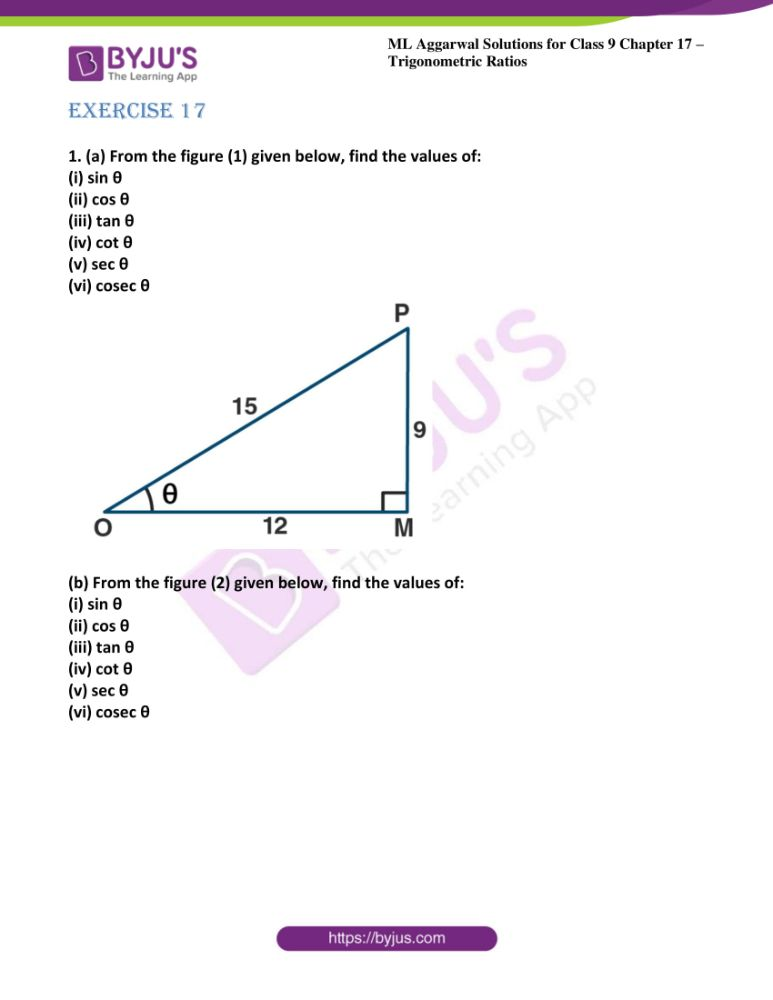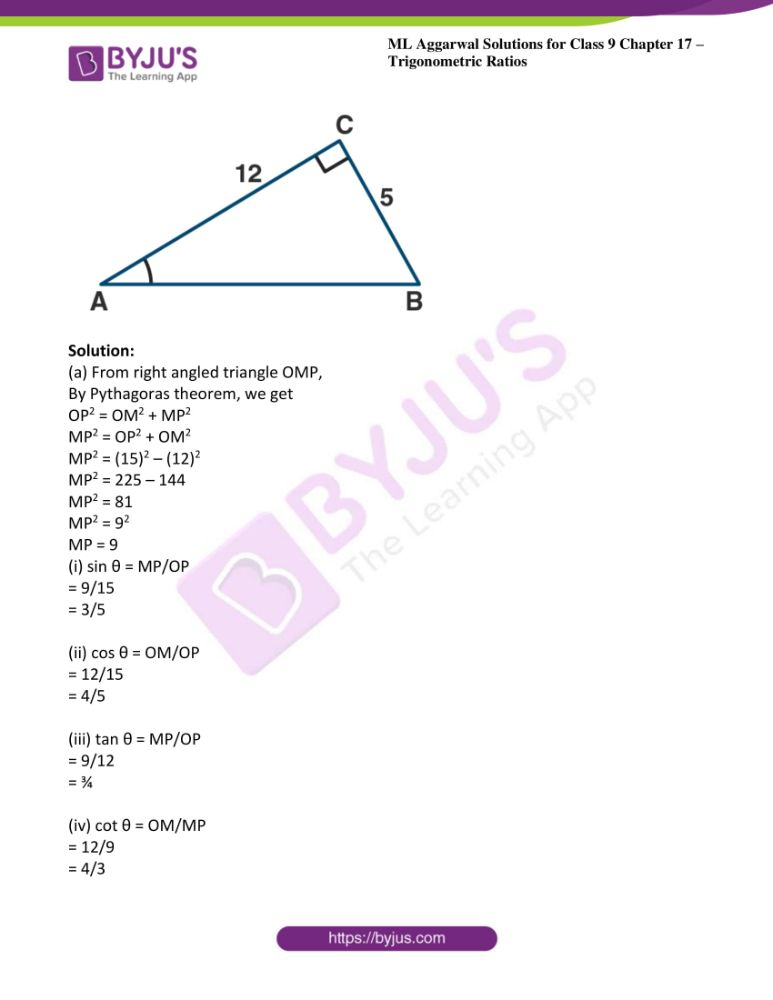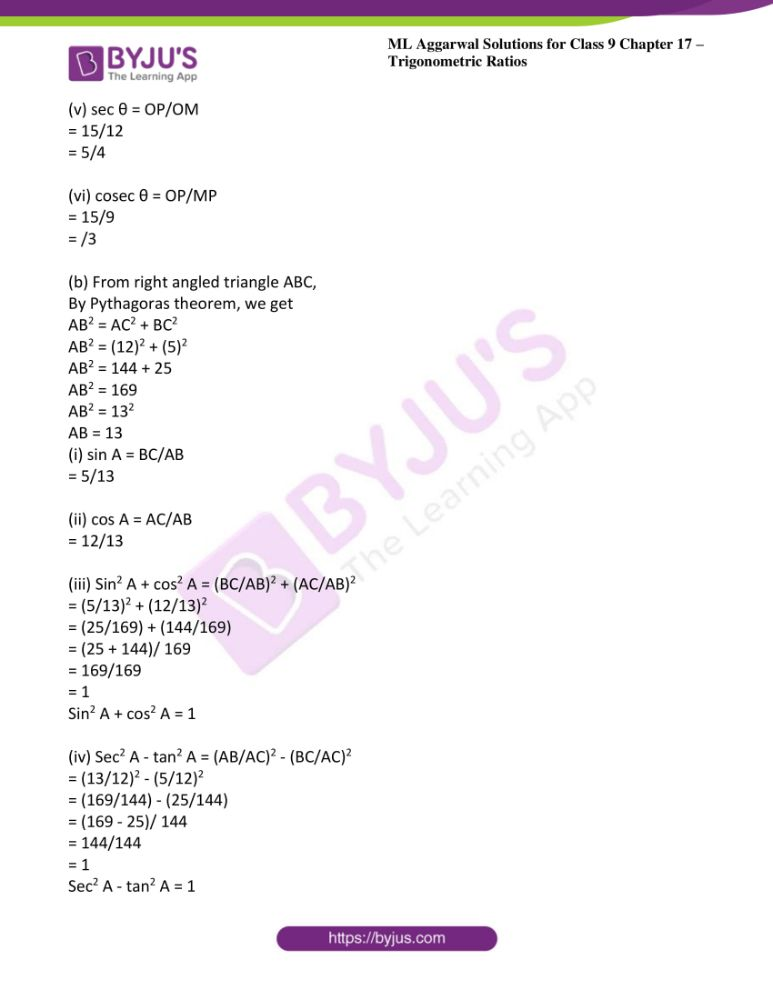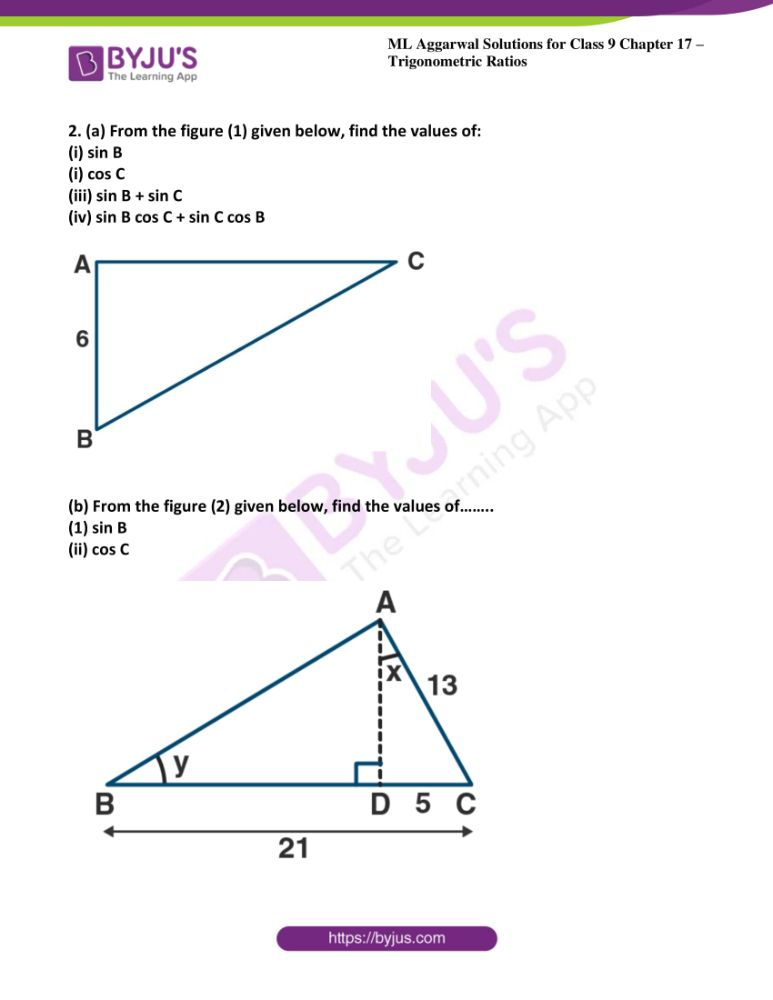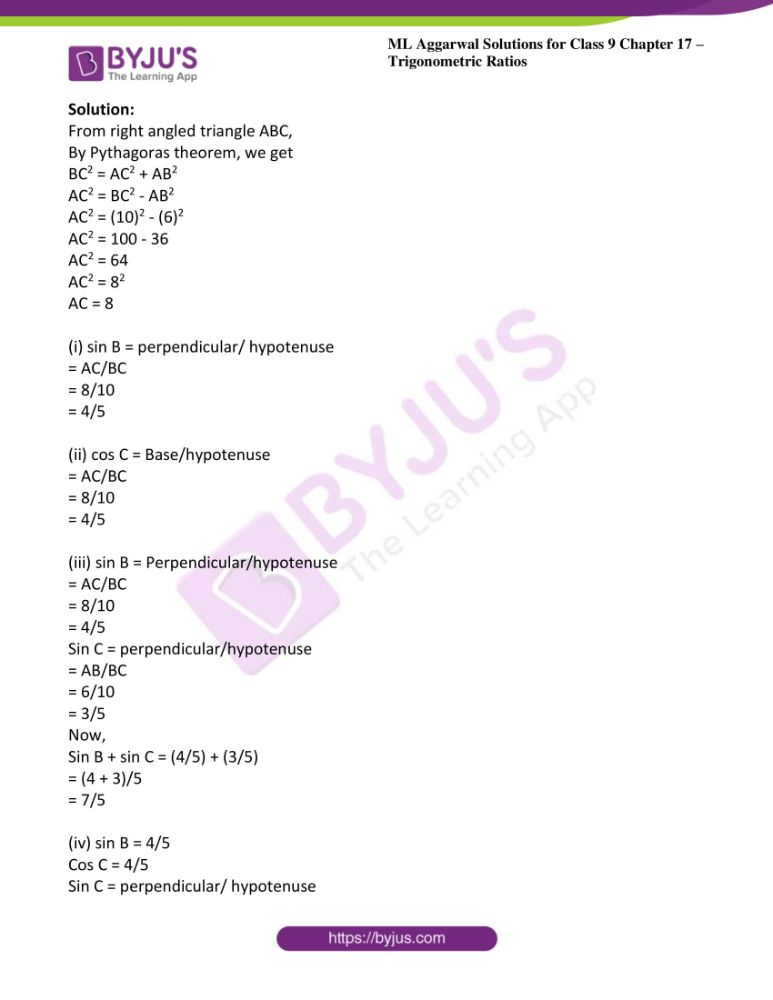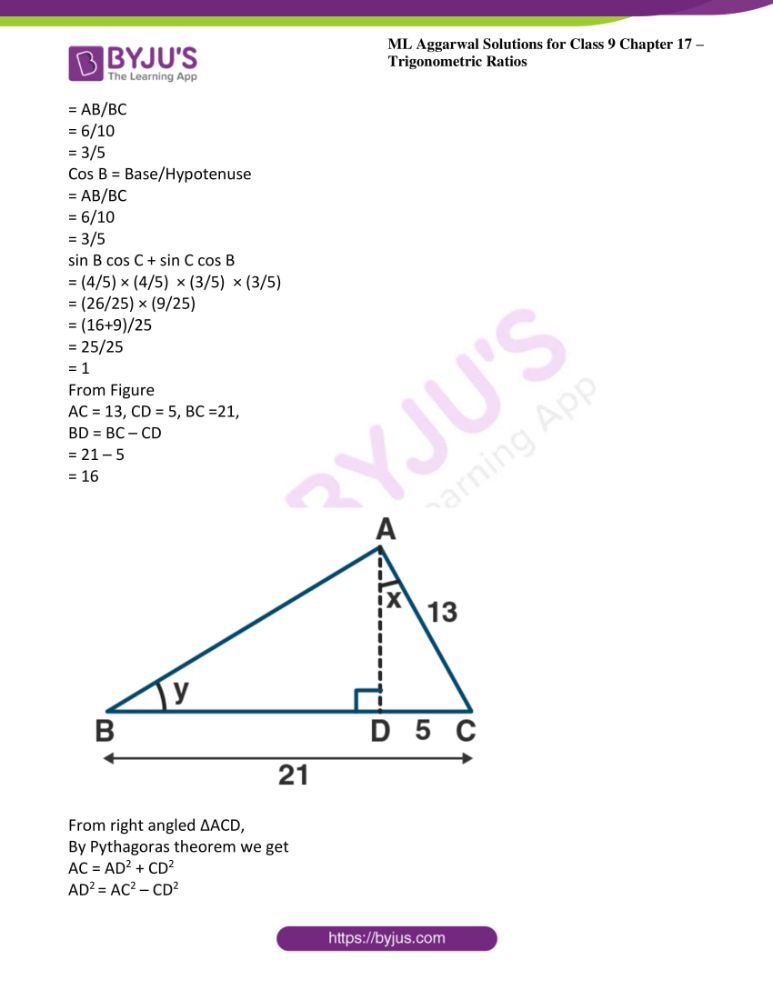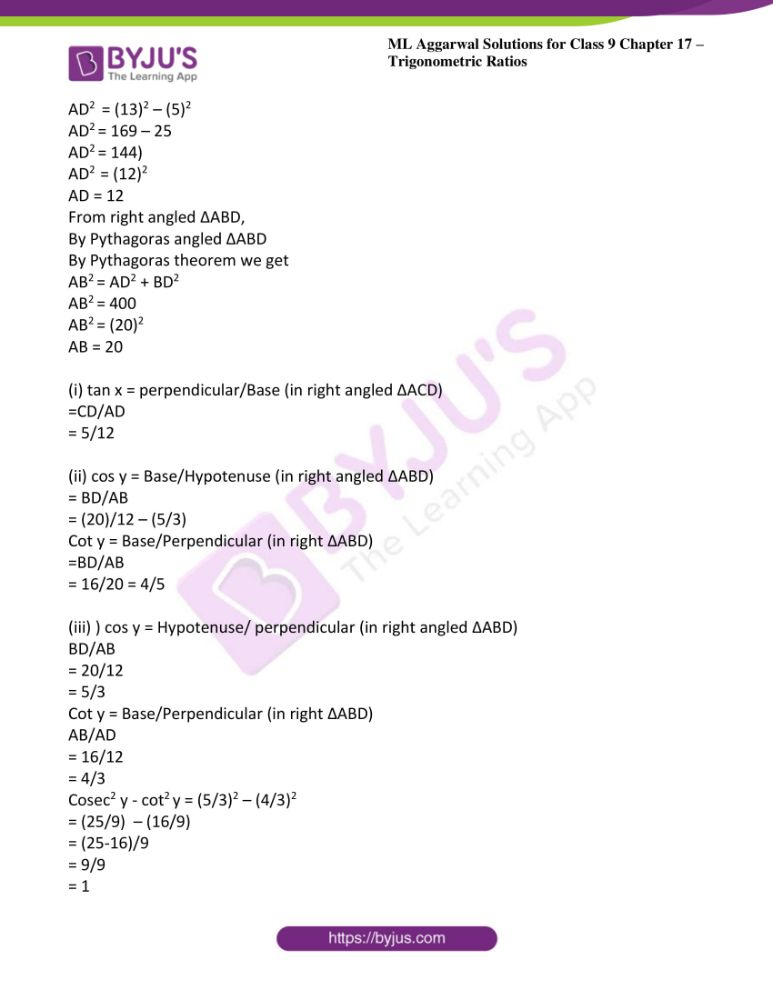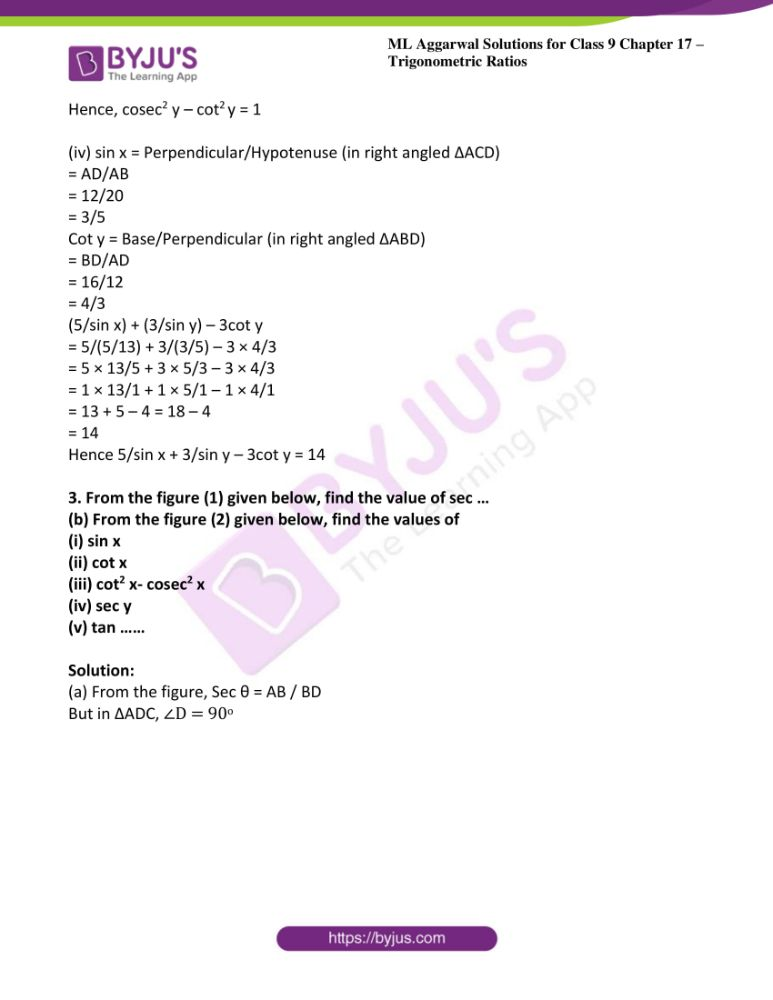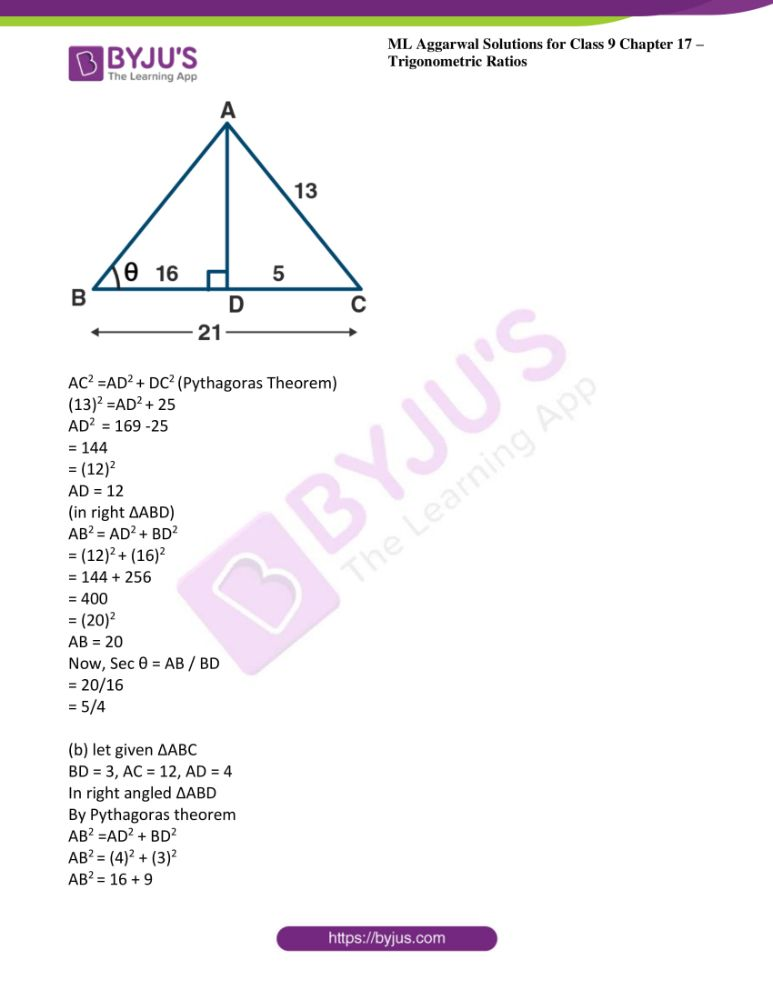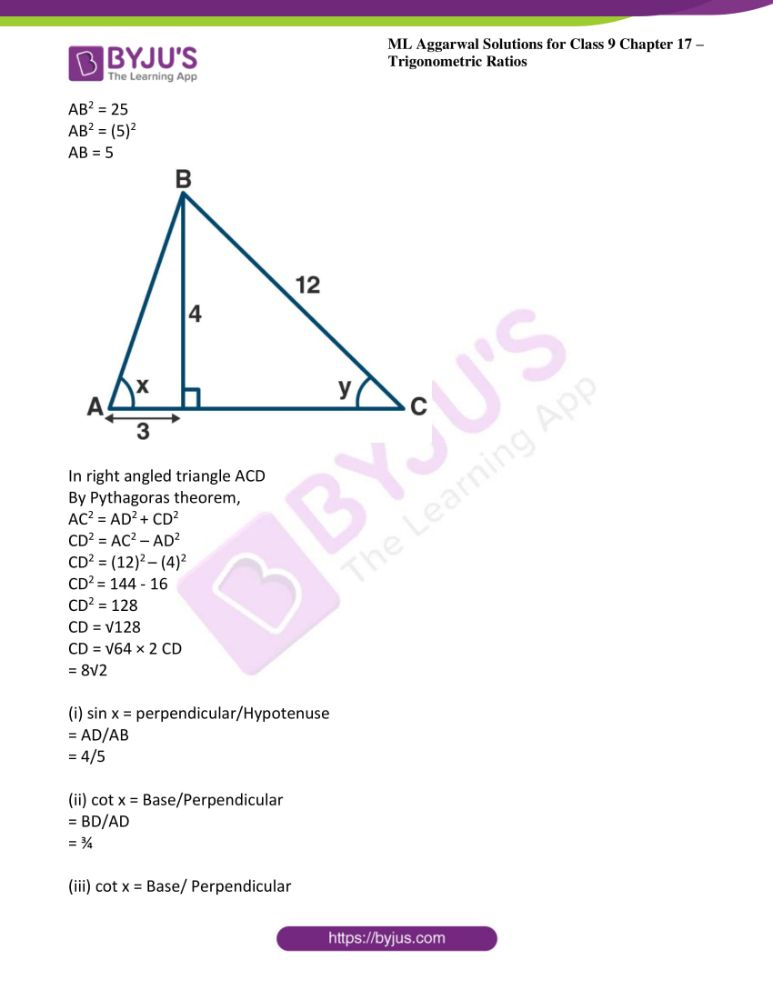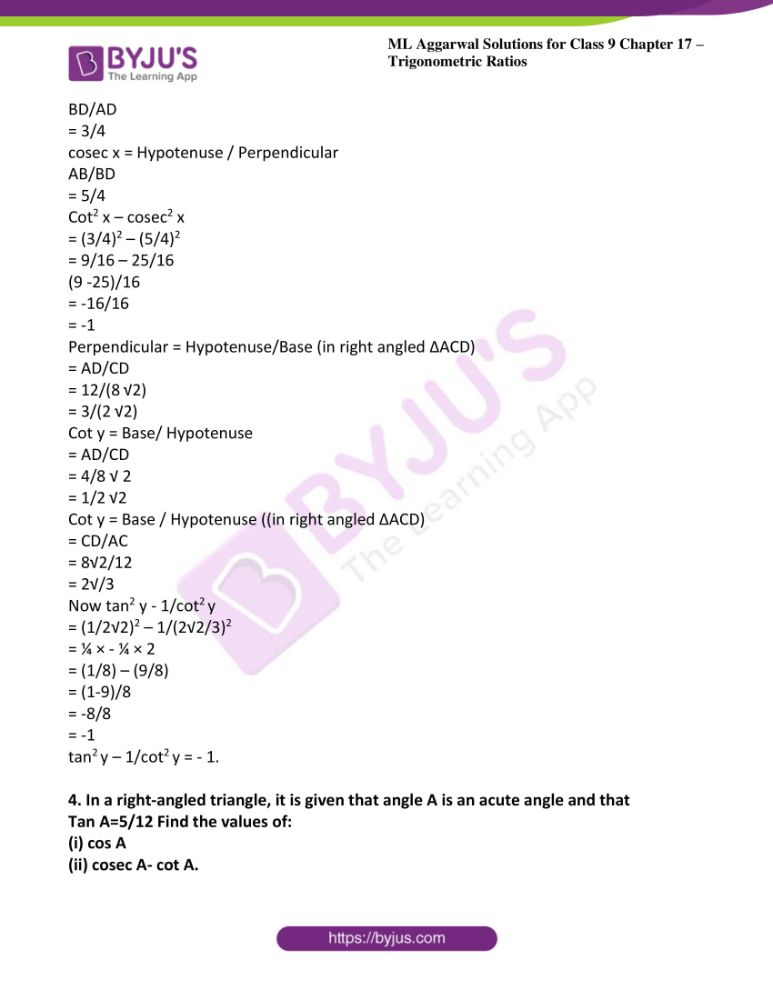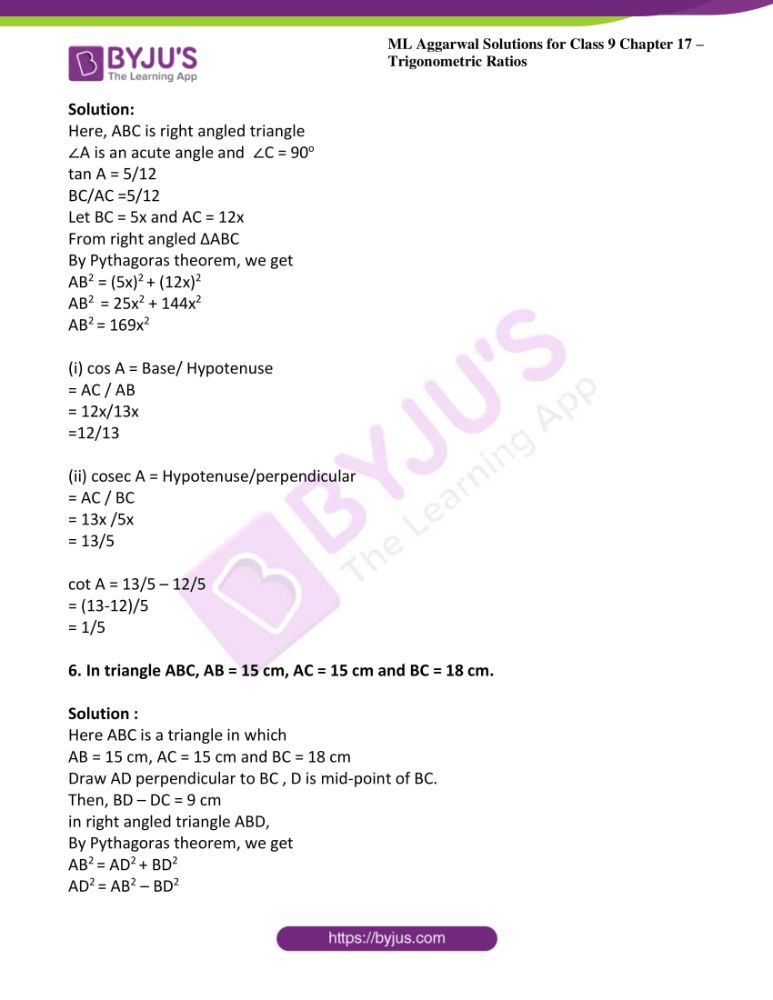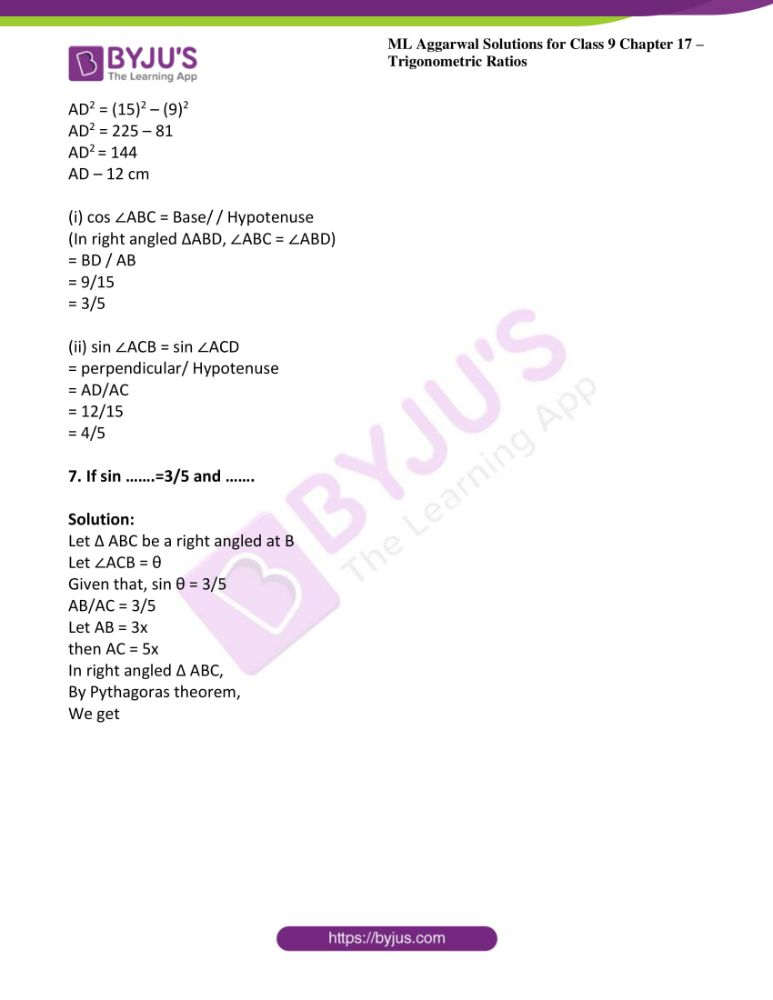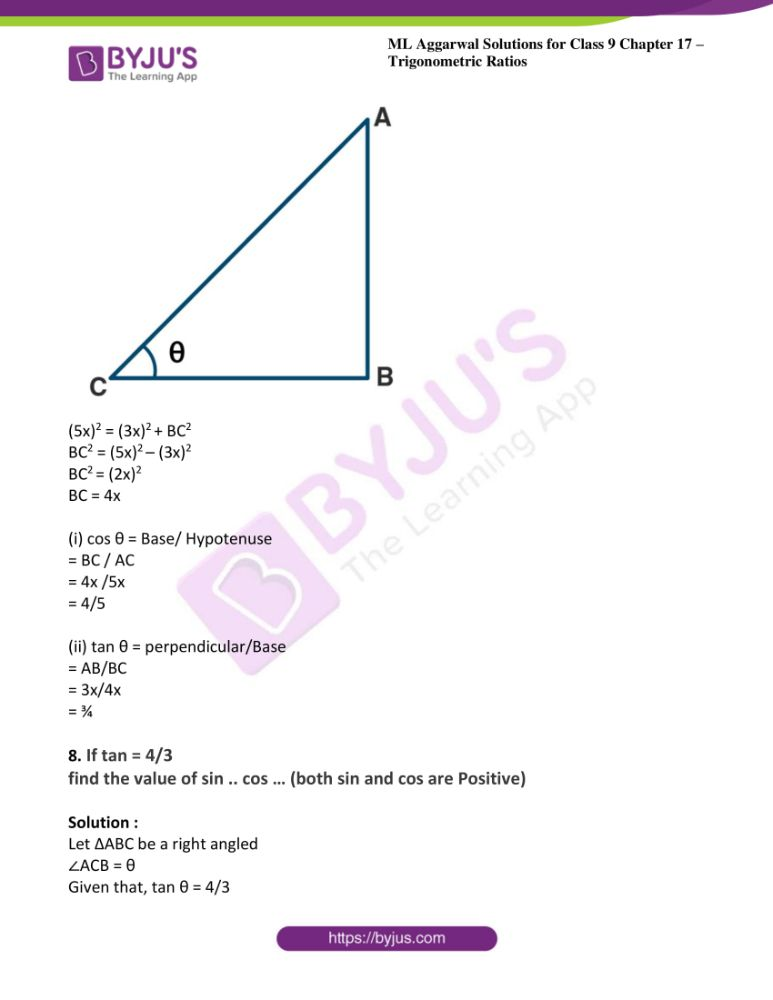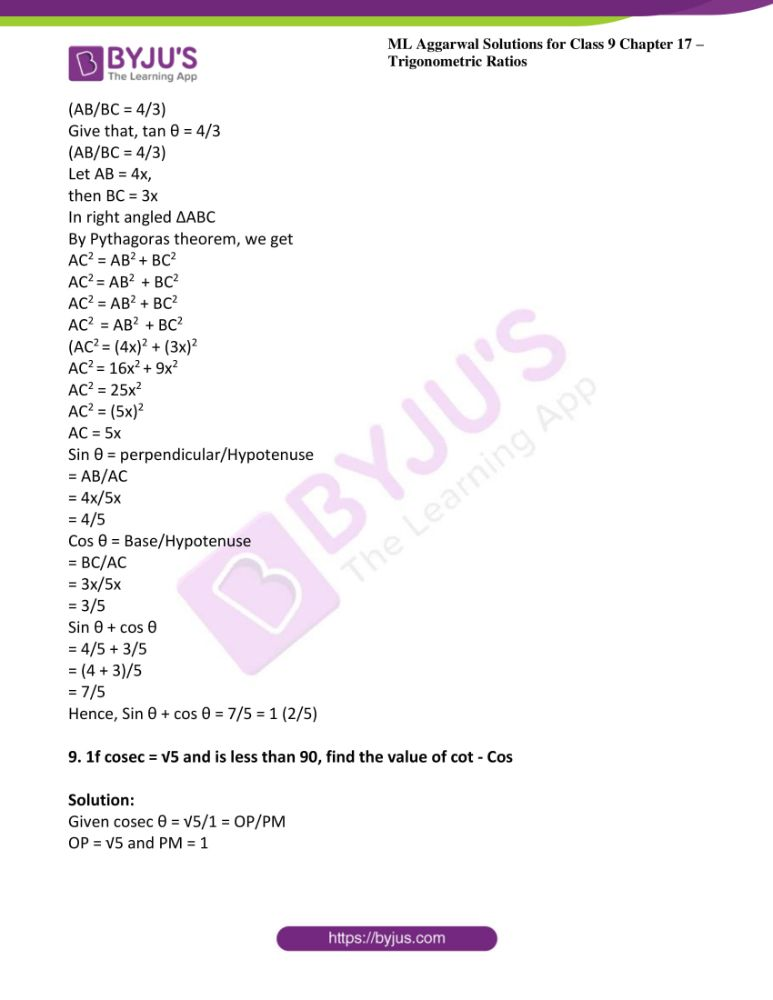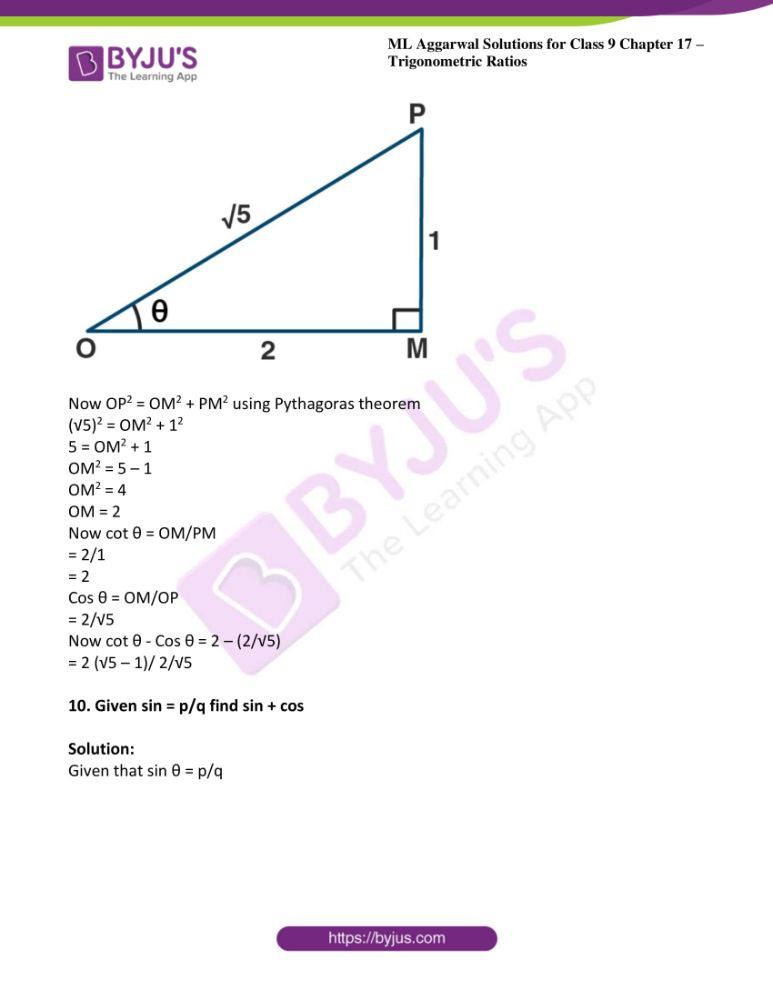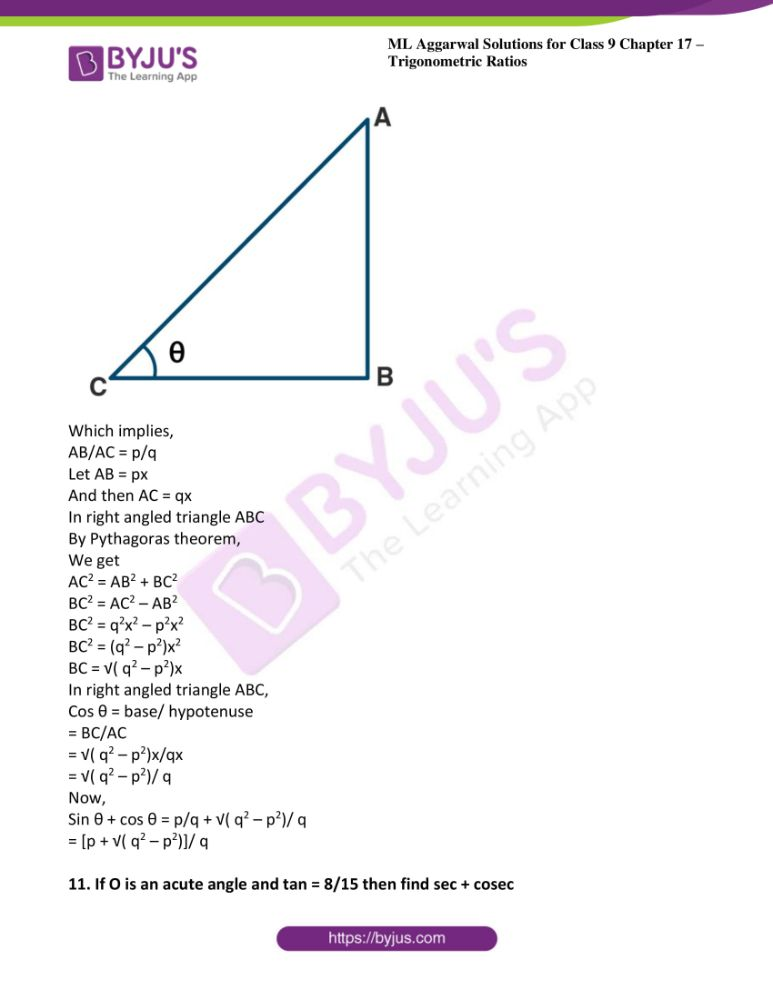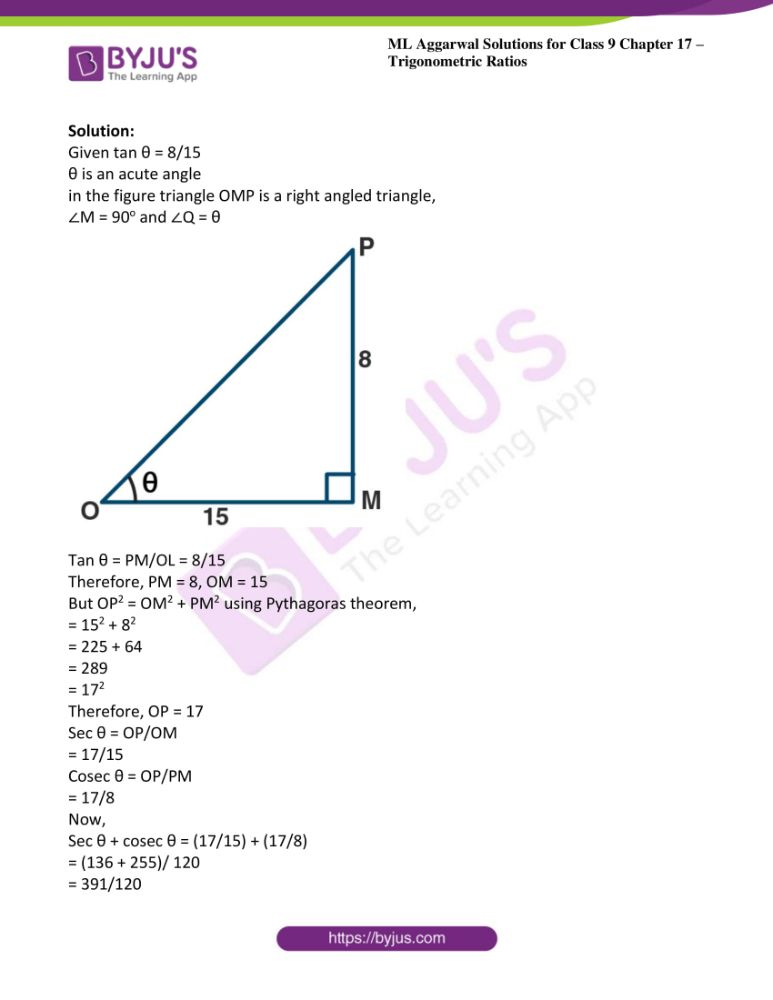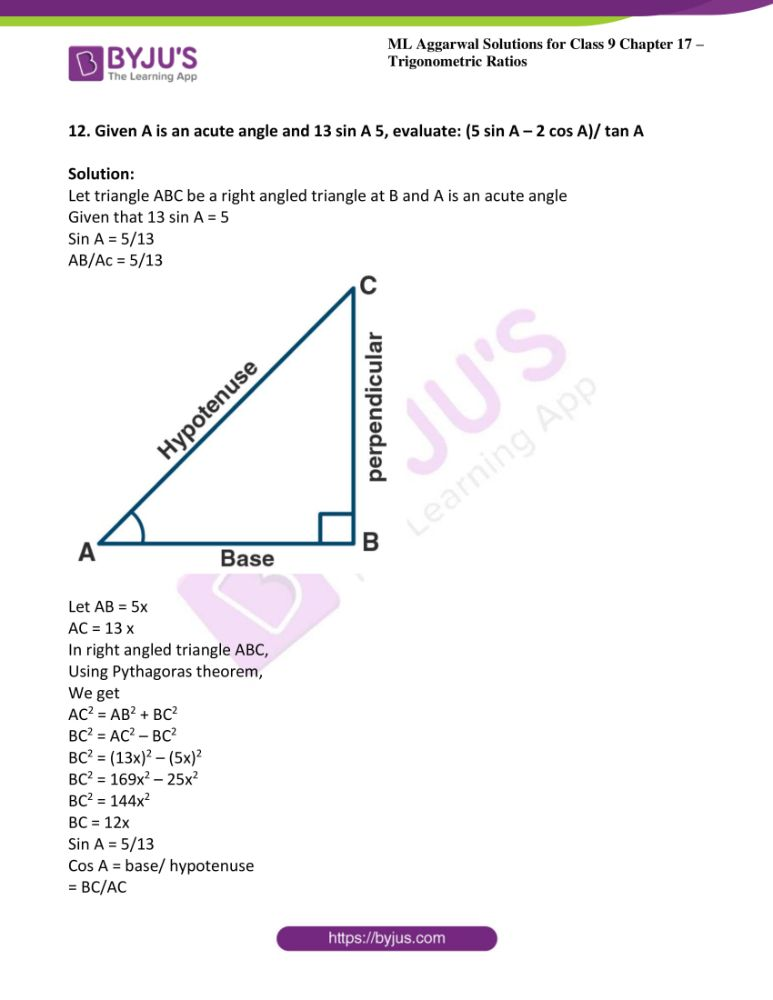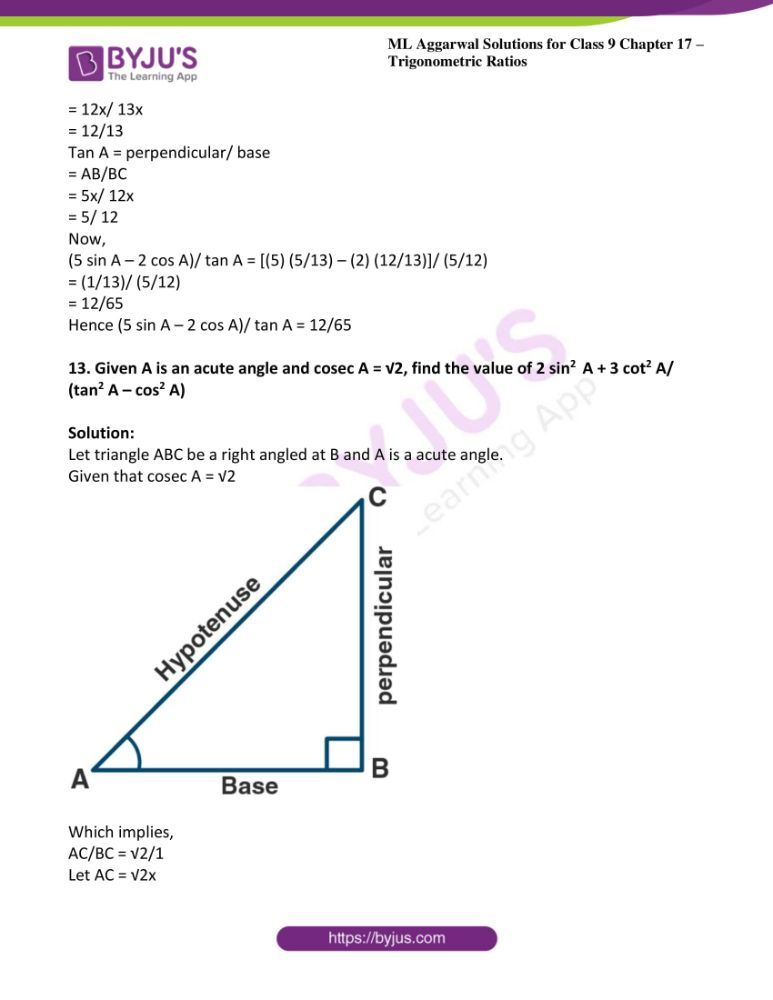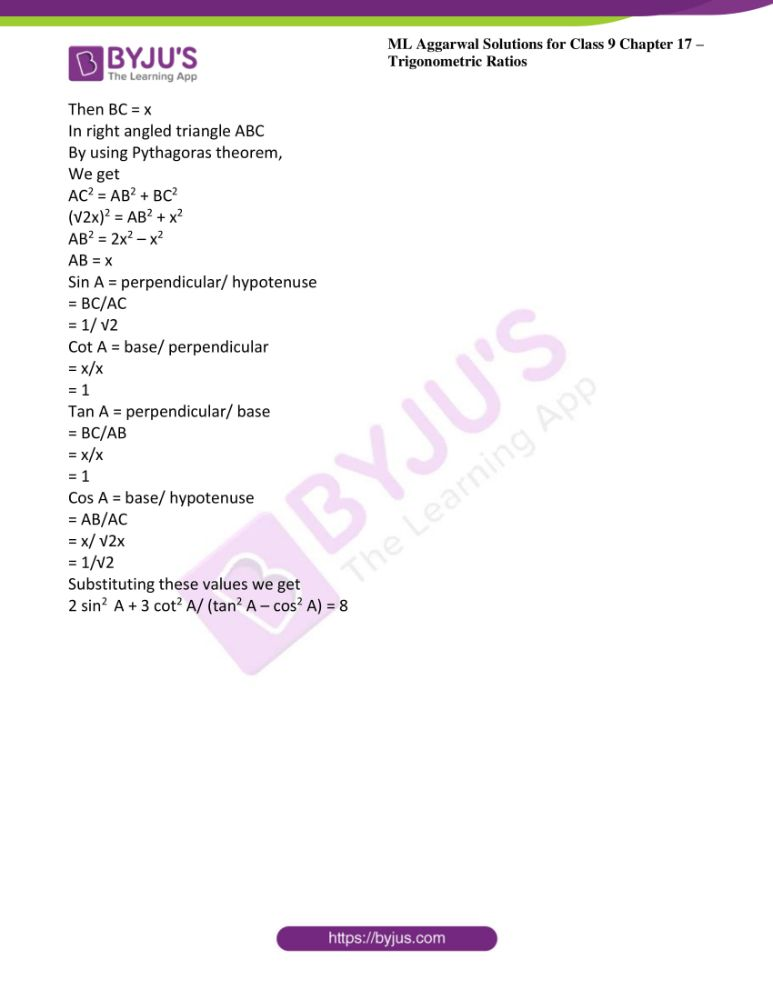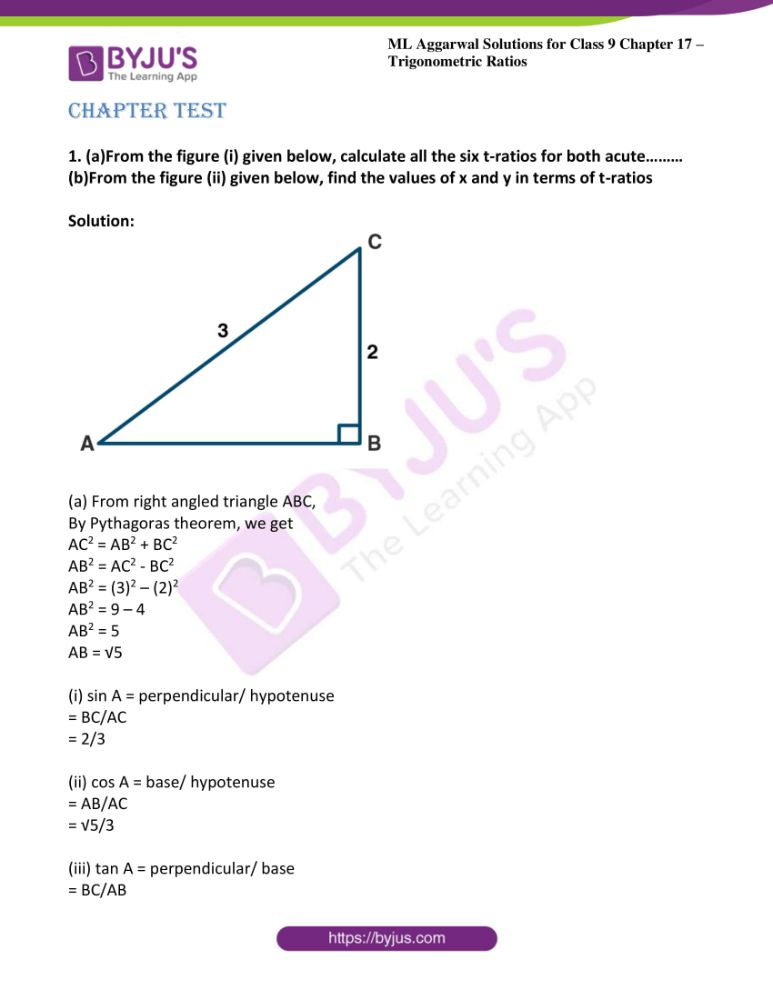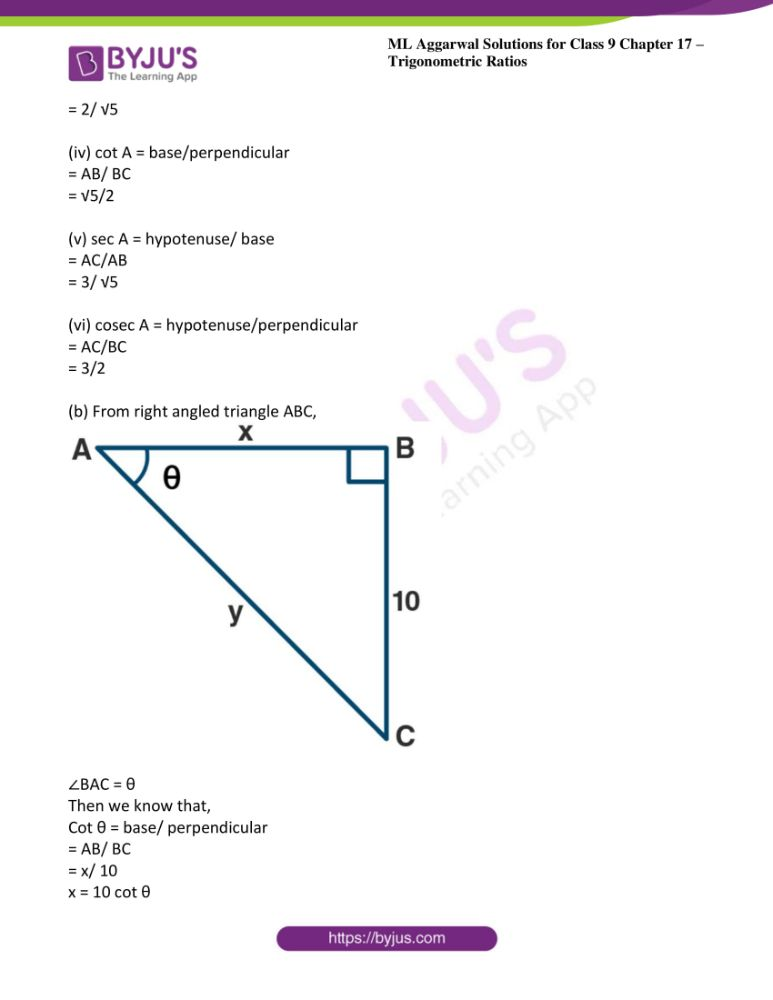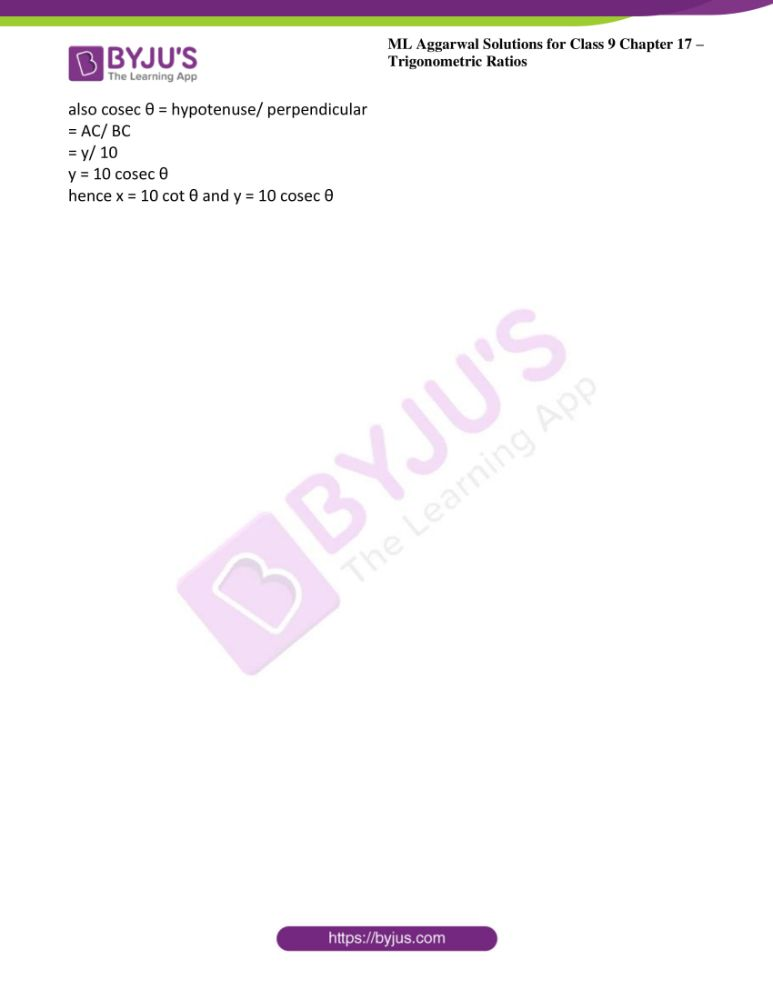### Access answers to ML Aggarwal Solutions for Class 9 Maths Chapter 17 – Trigonometric Ratios

Exercise 17

1. (a) From the figure (1) given below, find the values of:

(i) sin θ

(ii) cos θ

(iii) tan θ

(iv) cot θ

(v) sec θ

(vi) cosec θ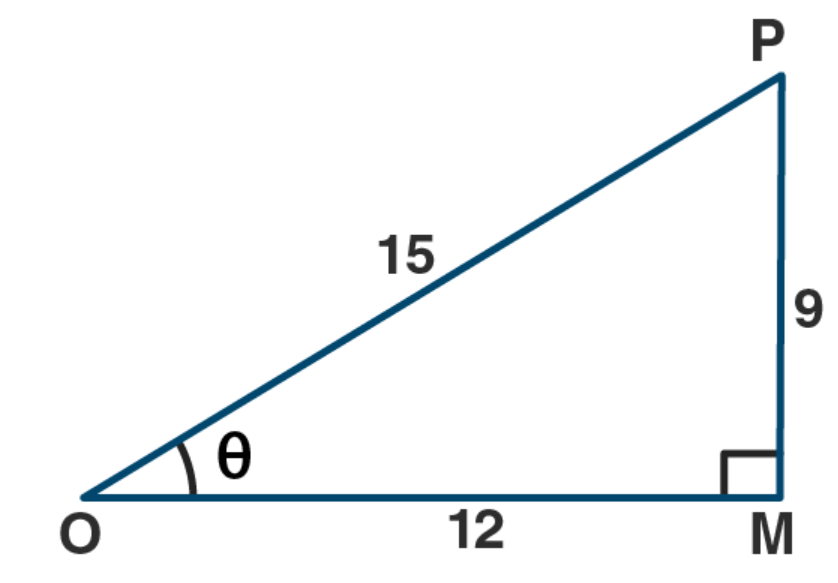(b) From the figure (2) given below, find the values of:

(i) sin θ

(ii) cos θ

(iii) tan θ

(iv) cot θ

(v) sec θ

(vi) cosec θ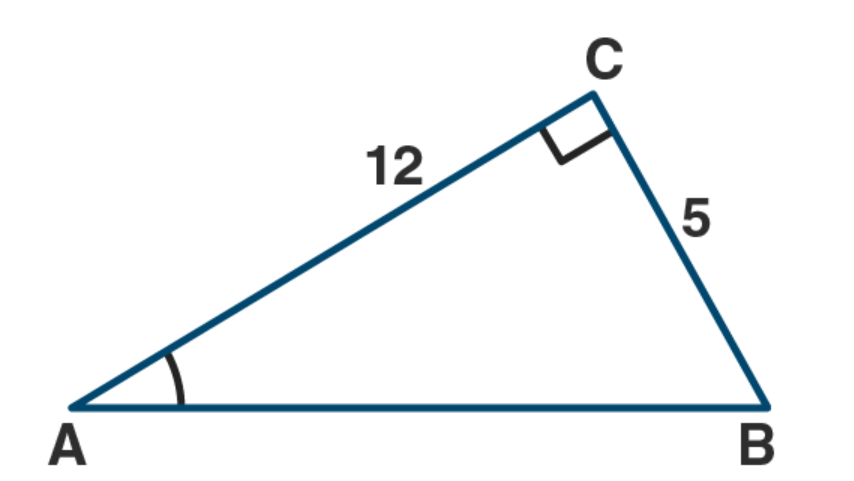Solution:

(a) From right angled triangle OMP,

By Pythagoras theorem, we get

OP2 = OM2 +
MP2

MP2 = OP2 + OM2

MP2 = (15)2 – (12)2

MP2 = 225 – 144

MP2 = 81

MP2 = 92

MP = 9

(i) sin θ = MP/OP

= 9/15

= 3/5

(ii) cos θ = OM/OP

= 12/15

= 4/5

(iii) tan θ = MP/OP

= 9/12

= ¾

(iv) cot θ = OM/MP

= 12/9

= 4/3

(v) sec θ = OP/OM

= 15/12

= 5/4

(vi) cosec θ = OP/MP

= 15/9

= /3

(b) From right angled triangle ABC,

By Pythagoras theorem, we get

AB2 = AC2 + BC2

AB2 = (12)2 + (5)2

AB2 = 144 + 25

AB2 = 169

AB2 = 132

AB = 13

(i) sin A = BC/AB

= 5/13

(ii) cos A = AC/AB

= 12/13

(iii)
Sin2 A + cos2 A = (BC/AB)2 + (AC/AB)2

= (5/13)2 + (12/13)2

= (25/169) + (144/169)

= (25 + 144)/ 169

= 169/169

= 1

Sin2 A + cos2 A = 1

(iv) Sec2 A – tan2 A = (AB/AC)2 – (BC/AC)2

= (13/12)2 – (5/12)2

= (169/144) – (25/144)

= (169 – 25)/ 144

= 144/144

= 1

Sec2 A – tan2 A = 1

2. (a) From the figure (1) given below, find the values of:
(i) sin B

(i) cos C

(iii) sin B + sin C

(iv) sin B cos C + sin C cos B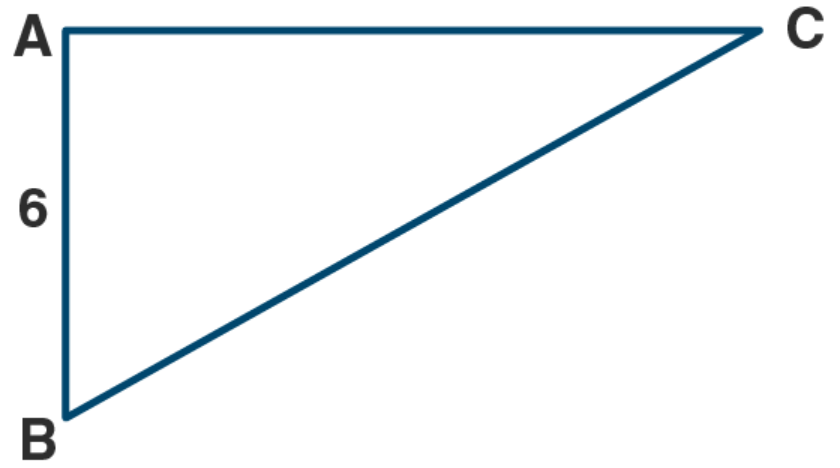(b) From the figure (2) given below, find the values of……..

(1) sin B

(ii) cos C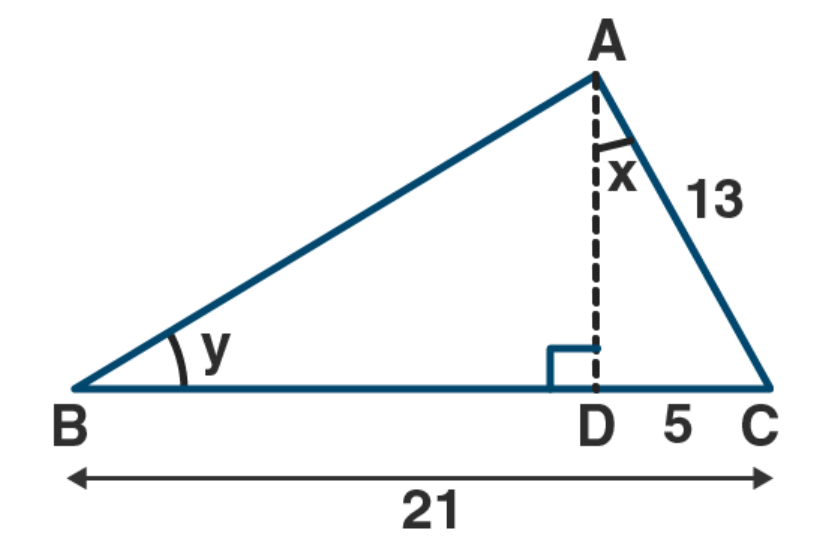Solution:

From right angled triangle ABC,

By Pythagoras theorem, we get

BC2 = AC2 + AB2

AC2 = BC2 – AB2

AC2 = (10)2 – (6)2

AC2 = 100 – 36

AC2 = 64

AC2 = 82

AC = 8

(i) sin B = perpendicular/ hypotenuse

= AC/BC

= 8/10

= 4/5

(ii) cos C = Base/hypotenuse

= AC/BC

= 8/10

= 4/5

(iii) sin B = Perpendicular/hypotenuse

= AC/BC

= 8/10

= 4/5

Sin C = perpendicular/hypotenuse

= AB/BC

= 6/10

= 3/5

Now,

Sin B + sin C = (4/5) + (3/5)

= (4 + 3)/5

= 7/5

(iv) sin B = 4/5

Cos C = 4/5

Sin C = perpendicular/ hypotenuse

= AB/BC

= 6/10

= 3/5

Cos B = Base/Hypotenuse

= AB/BC

= 6/10

= 3/5

sin B cos C + sin C cos B

= (4/5) × (4/5) × (3/5) × (3/5)

= (26/25) × (9/25)

= (16+9)/25

= 25/25

= 1

From Figure

AC = 13, CD = 5, BC =21,

BD = BC – CD

= 21 – 5

= 16From right angled ∆ACD,

By Pythagoras theorem we get

From right angled ∆ABD,

By Pythagoras angled ∆ABD

By Pythagoras theorem we get

AB2 = 400

AB2 = (20)2

AB = 20

(i) tan x = perpendicular/Base (in right angled ∆ACD)

= 5/12

(ii) cos y = Base/Hypotenuse (in right angled ∆ABD)

= BD/AB

= (20)/12 – (5/3)

Cot y = Base/Perpendicular (in right ∆ABD)

=BD/AB

= 16/20 = 4/5

(iii) ) cos y = Hypotenuse/ perpendicular (in right angled ∆ABD)

BD/AB

= 20/12

= 5/3

Cot y = Base/Perpendicular (in right ∆ABD)

= 16/12

= 4/3

Cosec2 y – cot2 y = (5/3)2 – (4/3)2

= (25/9) – (16/9)

= (25-16)/9

= 9/9

= 1

Hence, cosec2 y – cot2 y = 1

(iv) sin x = Perpendicular/Hypotenuse (in right angled ∆ACD)

= 12/20

= 3/5

Cot y = Base/Perpendicular (in right angled ∆ABD)

= 16/12

= 4/3

(5/sin x) + (3/sin y) – 3cot y

= 5/(5/13) + 3/(3/5) – 3 × 4/3

= 5 × 13/5 + 3 × 5/3 – 3 × 4/3

= 1 × 13/1 + 1 × 5/1 – 1 × 4/1

= 13 + 5 – 4 = 18 – 4

= 14

Hence 5/sin x + 3/sin y – 3cot y = 14

3. From the figure (1) given below, find the value of sec …
(b) From the figure (2) given below, find the values of
(i) sin x

(ii) cot x

(iii) cot2 x- cosec2 x

(iv) sec y

(v) tan ……

Solution:

(a) From the figure, Sec θ = AB / BD

But in ∆ADC, ∠D = 90o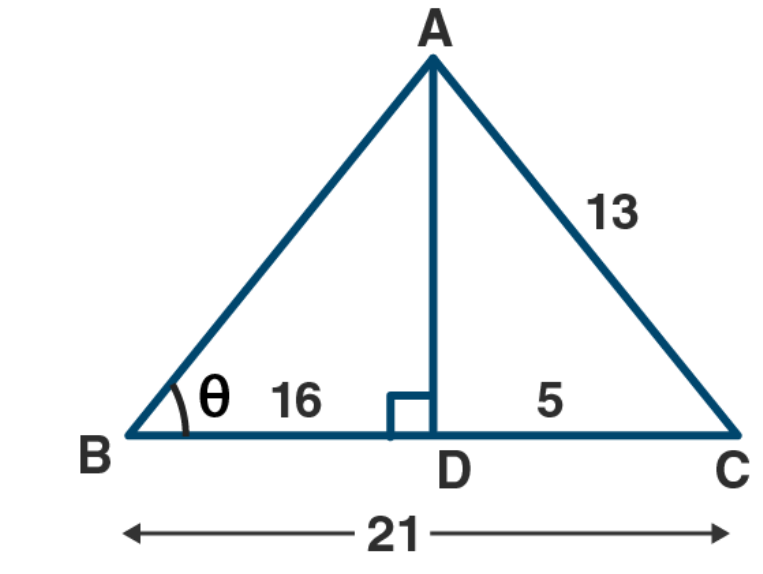AC2 =AD2 + DC2 (Pythagoras Theorem)

= 144

= (12)2

(in right ∆ABD)

= (12)2 + (16)2

= 144 + 256

= 400

= (20)2

AB = 20

Now, Sec θ = AB / BD

= 20/16

= 5/4

(b) let given ∆ABC

BD = 3, AC = 12, AD = 4

In right angled ∆ABD

By Pythagoras theorem

AB2 = (4)2 + (3)2

AB2 = 16 + 9

AB2 = 25

AB2 = (5)2

AB = 5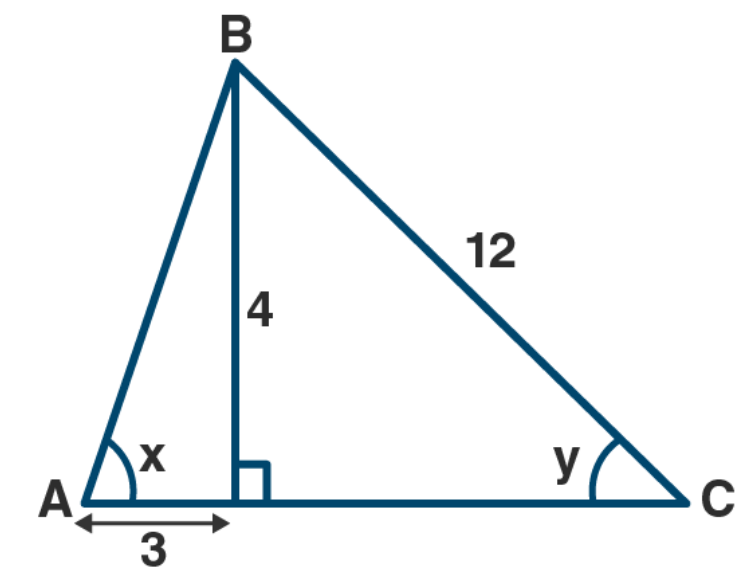In right angled triangle ACD

By Pythagoras theorem,

CD2 = (12)2 – (4)2

CD2 = 144 – 16

CD2 = 128

CD = √128

CD = √64 × 2 CD

= 8√2

(i) sin x = perpendicular/Hypotenuse

= 4/5

(ii) cot x = Base/Perpendicular

= ¾

(iii) cot x = Base/ Perpendicular

= 3/4

cosec x = Hypotenuse / Perpendicular

AB/BD

= 5/4

Cot2 x – cosec2 x

= (3/4)2 – (5/4)2

= 9/16 – 25/16

(9 -25)/16

= -16/16

= -1

Perpendicular = Hypotenuse/Base (in right angled ∆ACD)

= 12/(8 √2)

= 3/(2 √2)

Cot y = Base/ Hypotenuse

= 4/8 √ 2

= 1/2 √2

Cot y = Base / Hypotenuse ((in right angled ∆ACD)

= CD/AC

= 8√2/12

= 2√/3

Now tan2 y – 1/cot2 y

= (1/2√2)2 – 1/(2√2/3)2

= ¼ × – ¼ × 2

= (1/8) – (9/8)

= (1-9)/8

= -8/8

= -1

tan2 y – 1/cot2 y = – 1.

4. In a right-angled triangle, it is given that angle A is an acute angle and that
Tan A=5/12 Find the values of:
(i) cos A
(ii) cosec A- cot A.

Solution:

Here, ABC is right angled triangle

∠A is an acute angle and ∠C = 90o

tan A = 5/12

BC/AC =5/12

Let BC = 5x and AC = 12x

From right angled ∆ABC

By Pythagoras theorem, we get

AB2 = (5x)2 + (12x)2

AB2 = 25x2 + 144x2

AB2 = 169x2

(i) cos A = Base/ Hypotenuse

= AC / AB

= 12x/13x

=12/13

(ii) cosec A = Hypotenuse/perpendicular

= AC / BC

= 13x /5x

= 13/5

cot A = 13/5 – 12/5

= (13-12)/5

= 1/5

6. In triangle ABC, AB = 15 cm, AC = 15 cm and BC = 18 cm.

Solution :

Here ABC is a triangle in which

AB = 15 cm, AC = 15 cm and BC = 18 cm

Draw AD perpendicular to BC , D is mid-point of BC.

Then, BD – DC = 9 cm

in right angled triangle ABD,

By Pythagoras theorem, we get

(i) cos ∠ABC = Base/ / Hypotenuse

(In right angled ∆ABD, ∠ABC = ∠ABD)

= BD / AB

= 9/15

= 3/5

(ii) sin ∠ACB = sin ∠ACD

= perpendicular/ Hypotenuse

= 12/15

= 4/5

7. If sin …….=3/5 and …….

Solution:

Let ∆ ABC be a right angled at B

Let ∠ACB = θ

Given that, sin θ = 3/5

AB/AC = 3/5

Let AB = 3x

then AC = 5x

In right angled ∆ ABC,

By Pythagoras theorem,

We get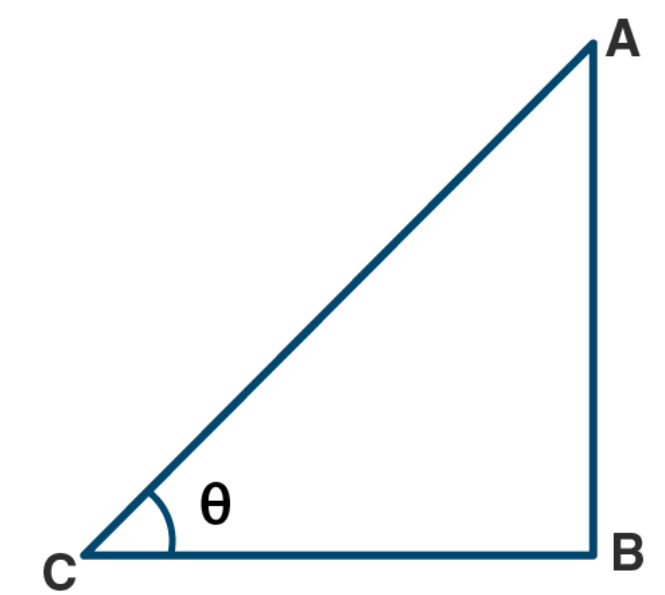(5x)2 = (3x)2 + BC2

BC2 = (5x)2 – (3x)2

BC2 = (2x)2

BC = 4x

(i) cos θ = Base/ Hypotenuse

= BC / AC

= 4x /5x

= 4/5

(ii) tan θ = perpendicular/Base

= AB/BC

= 3x/4x

= ¾

8. If tan = 4/3

find the value of sin .. cos … (both sin and cos are Positive)

Solution :

Let ∆ABC be a right angled

∠ACB = θ

Given that, tan θ = 4/3

(AB/BC = 4/3)

Give that, tan θ = 4/3

(AB/BC = 4/3)

Let AB = 4x,

then BC = 3x

In right angled ∆ABC

By Pythagoras theorem, we get

AC2 = AB2 + BC2

AC2 = AB2 + BC2

AC2 = AB2 + BC2

AC2 = AB2 + BC2

(AC2 = (4x)2 + (3x)2

AC2 = 16x2 + 9x2

AC2 = 25x2

AC2 = (5x)2

AC = 5x

Sin θ = perpendicular/Hypotenuse

= AB/AC

= 4x/5x

= 4/5

Cos θ = Base/Hypotenuse

= BC/AC

= 3x/5x

= 3/5

Sin θ + cos θ

= 4/5 + 3/5

= (4 + 3)/5

= 7/5

Hence, Sin θ + cos θ = 7/5 = 1 (2/5)

9. 1f cosec = √5 and is less than 90, find the value of cot – Cos

Solution:

Given cosec θ = √5/1 = OP/PM

OP = √5 and PM = 1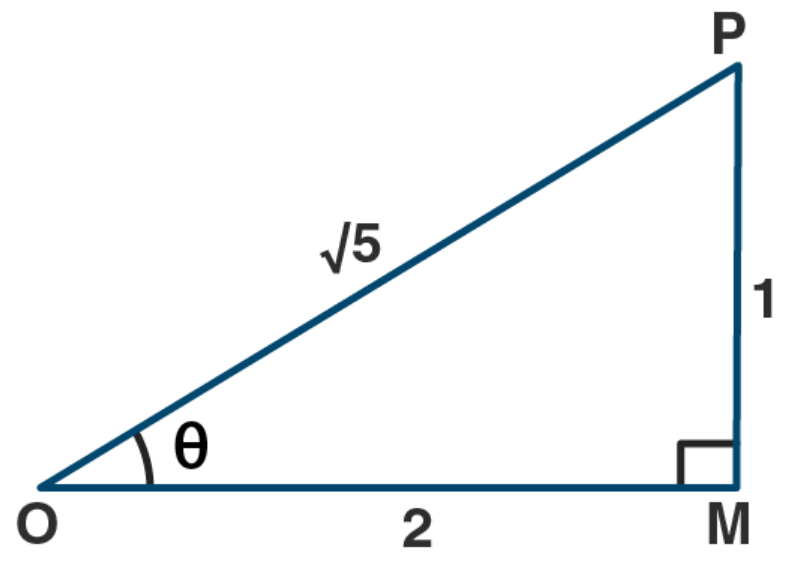Now OP2 = OM2 + PM2 using Pythagoras theorem

(√5)2 = OM2 + 12

5 = OM2 + 1

OM2 = 5 – 1

OM2 = 4

OM = 2

Now cot θ = OM/PM

= 2/1

= 2

Cos θ = OM/OP

= 2/√5

Now cot θ – Cos θ = 2 – (2/√5)

= 2 (√5 – 1)/ 2/√5

10. Given sin = p/q find sin + cos

Solution:

Given that sin θ = p/qWhich implies,

AB/AC = p/q

Let AB = px

And then AC = qx

In right angled triangle ABC

By Pythagoras theorem,

We get

AC2 = AB2 + BC2

BC2 = AC2 – AB2

BC2 = q2x2 – p2x2

BC2 = (q2 – p2)x2

BC = √( q2 – p2)x

In right angled triangle ABC,

Cos θ = base/ hypotenuse

= BC/AC

= √( q2 – p2)x/qx

= √( q2 – p2)/ q

Now,

Sin θ + cos θ = p/q + √( q2 – p2)/ q

= [p + √( q2 – p2)]/ q

11. If O is an acute angle and tan = 8/15 then find sec + cosec

Solution:

Given tan θ = 8/15

θ is an acute angle

in the figure triangle OMP is a right angled triangle,

∠M = 90o and ∠Q = θ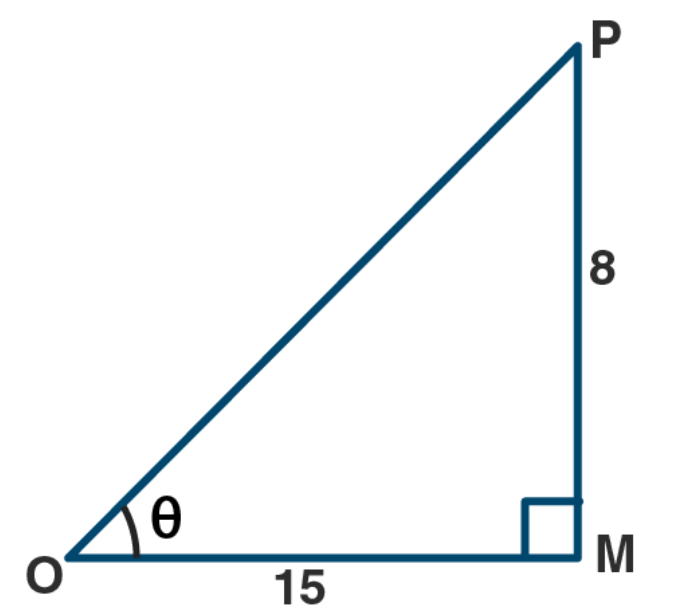Tan
θ = PM/OL = 8/15

Therefore, PM = 8, OM = 15

But OP2 = OM2 + PM2 using Pythagoras theorem,

= 152 + 82

= 225 + 64

= 289

= 172

Therefore, OP = 17

Sec θ = OP/OM

= 17/15

Cosec θ = OP/PM

= 17/8

Now,

Sec θ + cosec θ = (17/15) + (17/8)

= (136 + 255)/ 120

= 391/120

12. Given A is an acute angle and 13 sin A 5, evaluate: (5 sin A – 2 cos A)/ tan A

Solution:

Let triangle ABC be a right angled triangle at B and A is an acute angle

Given that 13 sin A = 5

Sin A = 5/13

AB/Ac = 5/13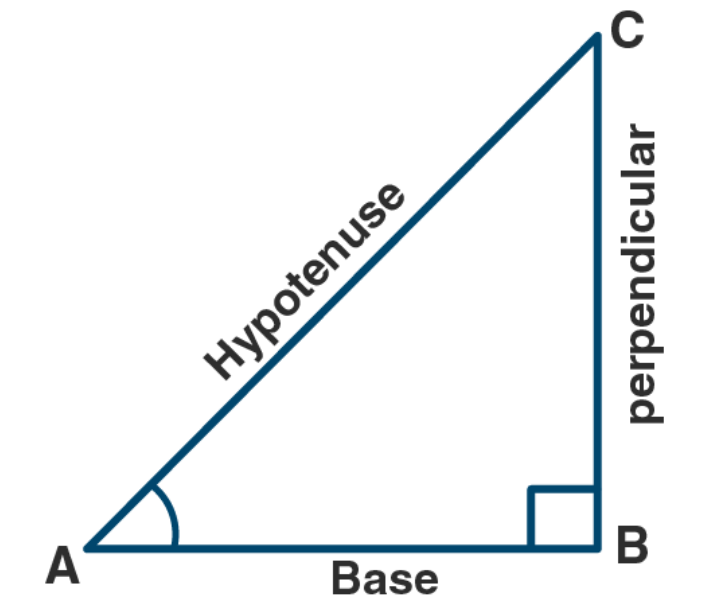Let AB = 5x

AC = 13 x

In right angled triangle ABC,

Using Pythagoras theorem,

We get

AC2 = AB2 + BC2

BC2 = AC2 – BC2

BC2 = (13x)2 – (5x)2

BC2 = 169x2 – 25x2

BC2 = 144x2

BC = 12x

Sin A = 5/13

Cos A = base/ hypotenuse

= BC/AC

= 12x/ 13x

= 12/13

Tan A = perpendicular/ base

= AB/BC

= 5x/ 12x

= 5/ 12

Now,

(5 sin A – 2 cos A)/ tan A = [(5) (5/13) – (2) (12/13)]/ (5/12)

= (1/13)/ (5/12)

= 12/65

Hence (5 sin A – 2 cos A)/ tan A = 12/65

13. Given A is an acute angle and cosec A = √2, find the value of 2 sin2 A + 3 cot2 A/ (tan2 A – cos2 A)

Solution:

Let triangle ABC be a right angled at B and A is a acute angle.

Given that cosec A = √2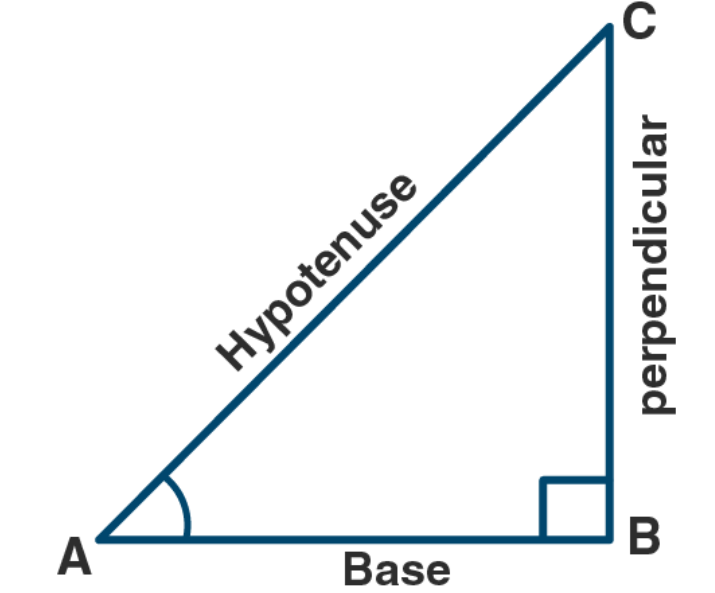Which implies,

AC/BC = √2/1

Let AC = √2x

Then BC = x

In right angled triangle ABC

By using Pythagoras theorem,

We get

AC2 = AB2 + BC2

(√2x)2 = AB2 + x2

AB2 = 2x2 – x2

AB = x

Sin A = perpendicular/ hypotenuse

= BC/AC

= 1/ √2

Cot A = base/ perpendicular

= x/x

= 1

Tan A = perpendicular/ base

= BC/AB

= x/x

= 1

Cos A = base/ hypotenuse

= AB/AC

= x/ √2x

= 1/√2

Substituting these values we get

2 sin2 A + 3 cot2 A/ (tan2 A – cos2 A) = 8

Chapter test

1. (a)From the figure (i) given below, calculate all the six t-ratios for both acute………

(b)From the figure (ii) given below, find the values of x and y in terms of t-ratios

Solution: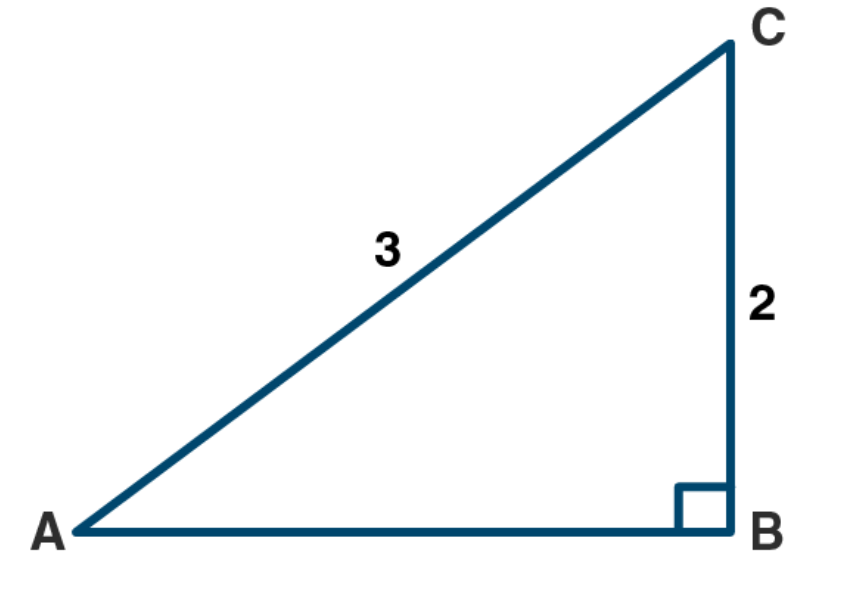(a) From right angled triangle ABC,

By Pythagoras theorem, we get

AC2 = AB2 + BC2

AB2 = AC2 – BC2

AB2 = (3)2 – (2)2

AB2 = 9 – 4

AB2 = 5

AB = √5

(i) sin A = perpendicular/ hypotenuse

= BC/AC

= 2/3

(ii) cos A = base/ hypotenuse

= AB/AC

= √5/3

(iii) tan A = perpendicular/ base

= BC/AB

= 2/ √5

(iv) cot A = base/perpendicular

= AB/ BC

= √5/2

(v) sec A = hypotenuse/ base

= AC/AB

= 3/ √5

(vi) cosec A = hypotenuse/perpendicular

= AC/BC

= 3/2

(b) From right angled triangle ABC,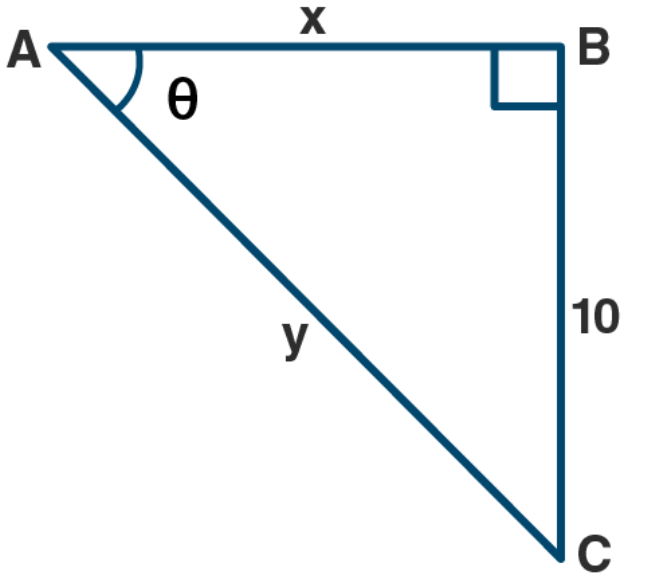∠BAC = θ

Then we know that,

Cot θ = base/ perpendicular

= AB/ BC

= x/ 10

x = 10 cot θ

also cosec θ = hypotenuse/ perpendicular

= AC/ BC

= y/ 10

y = 10 cosec θ

hence x = 10 cot θ and y = 10 cosec θ